GSAK (Geocaching Swiss Army Knife)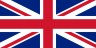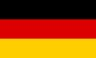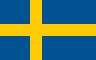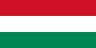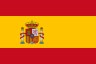Contents - Index

SqlGetClose (command)

SqlGetClose [Handle=n]

Iterating through a table via SqlGet=Handle, places a "lock" on the table which must be released. When you reach the end of the table (\$_SqlEoln = true) this lock is automatically released. However there may be times when you don't want to iterate though the whole table and you use the Break command to exit the loop. If you do exit the loop before \$_SqlEoln = true, then you must use SqlGetClose to make sure you close the table and release the lock.

Handle - this is the corresponding handle number (0 to 4) used in the Sqlite() function with the option SqlGet=Handle. If the Handle= parameter is omitted then the default handle of 0 is used.

Example:

 # list the names of the first 3 caches in the grid \$Data = "" \$x = 0 \$status = sqlite("sql",\$_SqlGrid, "sqlget=0") while not(\$_sqleol0)   \$x = \$x + 1   \$data = \$data + sqlget("name",0) + \$_CrLf   if \$x = 3     SqlGetClose Handle=0     Break   EndIf   sqlnext Handle=0 Endwhile cancel msg=\$data

As the default handle is 0, the Handle for SqlGetClose,SqlNext,SqlGet and \$_SqlEol is not really required. So for brevity, the previous example can also be coded as:

 # list the names of the first 3 caches in the grid \$Data = "" \$x = 0 \$status = sqlite("sql",\$_SqlGrid, "sqlget=0") while not(\$_sqleol)   \$x = \$x + 1   \$data = \$data + sqlget("name") + \$_CrLf   if \$x = 3     SqlGetClose     Break   EndIf   sqlnext Endwhile cancel msg=\$data